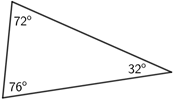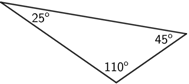# Oblique

The term oblique refers to being neither parallel nor perpendicular.

The term is used to describe many geometric objects such as the ones below.

## Oblique angle

An oblique angle is an angle that does not measure 90° or some multiple of 90°. If two intersecting lines are not perpendicular, oblique angles are formed. The lines are oblique lines.

## Oblique triangles

An oblique triangle contains no interior angle that is a right angle. An oblique triangle can be further categorized as either an acute triangle or an obtuse triangle.

Acute triangleObtuse TriangleEach angle has a measure less than 90°.An obtuse triangle contains one angle greater than 90°.

## Oblique prisms

An oblique prism is a prism whose lateral faces are not perpendicular to its bases.

## Oblique cylinder

Like the oblique prism, the sides of an oblique cylinder are not perpendicular to its circular bases.

## Oblique pictorials

An oblique pictorial describes the result of drawing a representation of a 3D figure on a 2D plane, such as a piece of paper.

As an example, draw a cube on a piece of paper, as shown below. First, a square face is drawn as it would look head-on. Then, four parallel line segments are drawn at 45° from each vertex of the square, forming the other faces of the cube, and giving it a 3D view.